• 2． 哈夫曼算法的应用 (1) 假设文档内容从文件读取； (2) 设计哈夫曼算法的存储结构； (3) 设计哈夫曼编码和解码算法； (4) 分析算法的时间复杂度
• ## 5.哈夫曼编码

千次阅读 2020-04-07 21:49:33
哈夫曼编码1.哈夫曼编码的原理2....在算法导论中，哈夫曼编码使用优先队列管理结点序列，如果优先队列使用最小堆来维护，这样哈夫曼编码算法时间复杂度为O(n*lgn)； 哈夫曼编码主要用于数据压缩，...


哈夫曼编码
1.哈夫曼编码的原理2.代码实现

1.哈夫曼编码的原理
构建哈夫曼树的步骤： 1.对于给定个m个权值，从中选出权值最小和次小的两个树，将其作为左右子树，其根节点的值为子树节点的值的和; 重复上面操作直到所有节点都并入树中;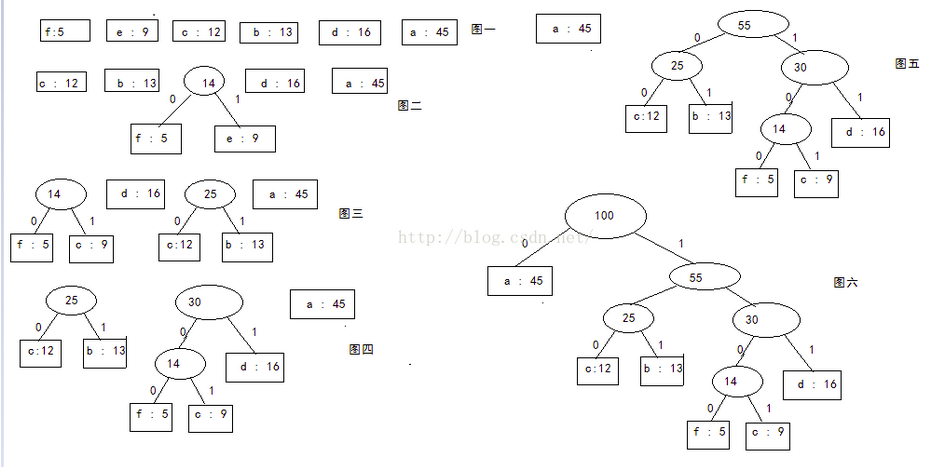在算法导论中，哈夫曼编码使用优先队列管理结点序列，如果优先队列使用最小堆来维护，这样哈夫曼编码算法时间复杂度为O(n*lgn)；

哈夫曼编码主要用于数据压缩，通常可以节省20%～90%的空间，具体的压缩依赖与数据的特性;

参考

注意：哈夫曼树的形态不是唯一的，但是它的带权路径长度WPL是唯一的; 最佳二叉树指的是哈夫曼树，但是哈夫曼树不一定就是平衡二叉树;

2.代码实现
/***************************************
目的：1.根据输入的字符代码集及其权值集，
构造赫夫曼树，输出各字符的赫夫曼编码
2.输入赫夫曼码序列，输出原始字符代码
作者：Dmego       时间：2016-11-11
****************************************/
#include<iostream>
#include<cstring>
#define MAX_MA 1000
#define MAX_ZF 100
using namespace std;

//哈夫曼树的储存表示
typedef struct
{
int weight;  //结点的权值
int parent, lchild, rchild;//双亲，左孩子，右孩子的下标
}HTNode,*HuffmanTree;  //动态分配数组来储存哈夫曼树的结点

//哈夫曼编码表的储存表示
typedef char **HuffmanCode;//动态分配数组存储哈夫曼编码

//返回两个双亲域为0且权值最小的点的下标
void Select(HuffmanTree HT, int n, int &s1, int &s2)
{
/*n代表HT数组的长度
*/

//前两个for循环找所有结点中权值最小的点（字符）
for (int i = 1; i <= n; i++)
{//利用for循环找出一个双亲为0的结点
if (HT[i].parent == 0)
{
s1 = i;//s1初始化为i
break;//找到一个后立即退出循环
}
}
for (int i = 1; i <= n; i++)
{/*利用for循环找到所有结点（字符）权值最小的一个
并且保证该结点的双亲为0*/
if (HT[i].weight < HT[s1].weight && HT[i].parent == 0)
s1 = i;
}
//后两个for循环所有结点中权值第二小的点（字符）
for (int i = 1; i <= n; i++)
{//利用for循环找出一个双亲为0的结点，并且不能是s1
if (HT[i].parent == 0 && i != s1)
{
s2 = i;//s2初始化为i
break;//找到一个后立即退出循环
}
}

for (int i = 1; i <= n; i++)
{/*利用for循环找到所有结点（字符）权值第二小的一个，
该结点满足不能是s1且双亲是0*/
if (HT[i].weight < HT[s2].weight && HT[i].parent == 0 && i!= s1)
s2 = i;
}

}

//构造哈夫曼树
void CreateHuffmanTree(HuffmanTree &HT, int n)
{
/*-----------初始化工作-------------------------*/
if (n <= 1)
return;
int m = 2 * n - 1;
HT = new HTNode[m + 1];
for (int i = 1; i <= m; ++i)
{//将1~m号单元中的双亲，左孩子，右孩子的下标都初始化为0
HT[i].parent = 0; HT[i].lchild = 0; HT[i].rchild = 0;
}
for (int i = 1; i <= n; ++i)
{
cin >> HT[i].weight;//输入前n个单元中叶子结点的权值
}
/*-----------创建工作---------------------------*/
int s1,s2;
for (int i = n + 1; i <= m; ++i)
{//通过n-1次的选择，删除，合并来构造哈夫曼树
Select(HT, i - 1, s1, s2);
/*cout << HT[s1].weight << " , " << HT[s2].weight << endl;*/
/*将s1,s2的双亲域由0改为i
(相当于把这两个结点删除了，这两个结点不再参与Select()函数)*/
HT[s1].parent = i;
HT[s2].parent = i;
//s1,与s2分别作为i的左右孩子
HT[i].lchild = s1;
HT[i].rchild = s2;
//结点i的权值为s1,s2权值之和
HT[i].weight = HT[s1].weight + HT[s2].weight;
}
}

//从叶子到根逆向求每个字符的哈夫曼编码，储存在编码表HC中
void CreatHuffmanCode(HuffmanTree HT, HuffmanCode &HC, int n)
{
HC = new char*[n + 1];//分配储存n个字符编码的编码表空间
char *cd = new char[n];//分配临时存储字符编码的动态空间
cd[n - 1] = '\0';//编码结束符
for (int i = 1; i <= n; i++)//逐个求字符编码
{
int start = n - 1;//start 开始指向最后，即编码结束符位置
int c = i;
int f = HT[c].parent;//f指向结点c的双亲
while (f != 0)//从叶子结点开始回溯，直到根结点
{
--start;//回溯一次，start向前指向一个位置
if (HT[f].lchild == c) cd[start] = '0';//结点c是f的左孩子，则cd[start] = 0;
else cd[start] = '1';//否则c是f的右孩子，cd[start] = 1
c = f;
f = HT[f].parent;//继续向上回溯
}
HC[i] = new char[n - start];//为第i个字符编码分配空间
strcpy(HC[i], &cd[start]);//把求得编码的首地址从cd[start]复制到HC的当前行中
}
delete cd;
}

//哈夫曼译码
void TranCode(HuffmanTree HT,char a[],char zf[],char b[],int n)
{
/*
HT是已经创建好的哈夫曼树
a[]用来传入二进制编码
b[]用来记录译出的字符
zf[]是与哈夫曼树的叶子对应的字符（叶子下标与字符下标对应）
n是字符个数，相当于zf[]数组得长度
*/

int q = 2*n-1;//q初始化为根结点的下标
int k = 0;//记录存储译出字符数组的下标
int i = 0;
for (i = 0; a[i] != '\0';i++)
{//for循环结束条件是读入的字符是结束符（二进制编码）
//此代码块用来判断读入的二进制字符是0还是1
if (a[i] == '0')
{/*读入0，把根结点(HT[q])的左孩子的下标值赋给q
下次循环的时候把HT[q]的左孩子作为新的根结点*/
q = HT[q].lchild;
}
else if (a[i] == '1')
{
q = HT[q].rchild;
}
//此代码块用来判断HT[q]是否为叶子结点
if (HT[q].lchild == 0 && HT[q].rchild == 0)
{/*是叶子结点，说明已经译出一个字符
该字符的下标就是找到的叶子结点的下标*/
b[k++] = zf[q];//把下标为q的字符赋给字符数组b[]
q = 2 * n - 1;//初始化q为根结点的下标
//继续译下一个字符的时候从哈夫曼树的根结点开始
}
}
/*译码完成之后，用来记录译出字符的数组由于没有结束符输出的
时候回报错，故紧接着把一个结束符加到数组最后*/
b[k] = '\0';
}
//菜单函数
{
cout << endl;
cout << "       ┏〓〓〓〓〓〓〓〓〓〓〓〓〓〓〓〓〓〓〓〓〓〓〓〓〓〓〓〓〓┓" << endl;
cout << "       ┃      ★★★★★★★哈夫曼编码与译码★★★★★★★        ┃" << endl;
cout << "       ┃                   1.  创建哈夫曼树                       ┃" << endl;
cout << "       ┃                   2.  进行哈夫曼编码                     ┃" << endl;
cout << "       ┃                   3.  进行哈夫曼译码                     ┃" << endl;
cout << "       ┃                   4.  退出程序                           ┃" << endl;
cout << "       ┗〓〓〓〓〓〓〓〓〓〓〓〓〓〓〓〓〓〓〓〓〓〓〓〓〓〓〓〓〓┛" << endl;
cout << "                       <><注意：空格字符用'- '代替><>" << endl;
cout << endl;
}
int main()
{
int falg;//记录要编码的字符个数
char a[MAX_MA];//储存输入的二进制字符
char b[MAX_ZF];//存储译出的字符
char zf[MAX_ZF];//储存要编码的字符
HuffmanTree HT = NULL;//初始化树为空数
HuffmanCode HC = NULL;//初始化编码表为空表
while (true)
{
int num;
cout << "<><请选择功能(1-创建 2-编码 3-译码 4-退出)><>: ";
cin >> num;
switch (num)
{
case 1 :
cout << "<><请输入字符个数><>:";
cin >> falg;
//动态申请falg个长度的字符数组，用来存储要编码的字符
/*char *zf = new char[falg];*/
cout << "<><请依次输入" << falg << "个字符:><>: ";
for (int i = 1; i <= falg; i++)
cin >> zf[i];
cout << "<><请依次输入" << falg << "个字符的权值><>: ";
CreateHuffmanTree(HT, falg);//调用创建哈夫曼树的函数
cout << endl;
cout << "<><创建哈夫曼成功！,下面是该哈夫曼树的参数输出><>：" << endl;
cout << endl;
cout << "结点i"<<"\t"<<"字符" << "\t" << "权值" << "\t" << "双亲" << "\t" << "左孩子" << "\t" << "右孩子" << endl;
for (int i = 1; i <= falg * 2 - 1; i++)
{
cout << i << "\t"<<zf[i]<< "\t" << HT[i].weight << "\t" << HT[i].parent << "\t" << HT[i].lchild << "\t" << HT[i].rchild << endl;
}
cout << endl;
break;
case 2:
CreatHuffmanCode(HT, HC, falg);//调用创建哈夫曼编码表的函数
cout << endl;
cout << "<><生成哈夫曼编码表成功！,下面是该编码表的输出><>：" << endl;
cout << endl;
cout << "结点i"<<"\t"<<"字符" << "\t" << "权值" << "\t" << "编码" << endl;
for (int i = 1; i <= falg; i++)
{
cout << i << "\t"<<zf[i]<< "\t" << HT[i].weight << "\t" << HC[i] << endl;
}
cout << endl;
break;
case 3:
cout << "<><请输入想要翻译的一串二进制编码><>：";
/*这样可以动态的直接输入一串二进制编码，
因为这样输入时最后系统会自动加一个结束符*/
cin >> a;
TranCode(HT, a, zf, b, falg);//调用译码的函数，
/*这样可以直接把数组b输出，因为最后有
在数组b添加输出时遇到结束符会结束输出*/
cout << endl;
cout << "<><译码成功！翻译结果为><>：" << b << endl;
cout << endl;
break;
case 4:
cout << endl;
cout << "<><退出成功！><>" << endl;
exit(0);
default:
break;
}
}

//-abcdefghijklmnopqrstuvwxyz
//186 64 13 22 32 103 21 15 47 57 1 5 32 20 57 63 15 1 48 51 80 23 8 18 1 16 1
//000101010111101111001111110001100100101011110110
return 0;
}


代码链接
展开全文• a.b.c.d.e 对应出现频率为4,6,11,13,15 用Python实现哈夫曼代码可参考：哈夫曼树的 Python 实现 或者：https://blog.csdn.net/qq_42098718/article/details/102809485
a.b.c.d.e 对应出现频率为4,6,11,13,15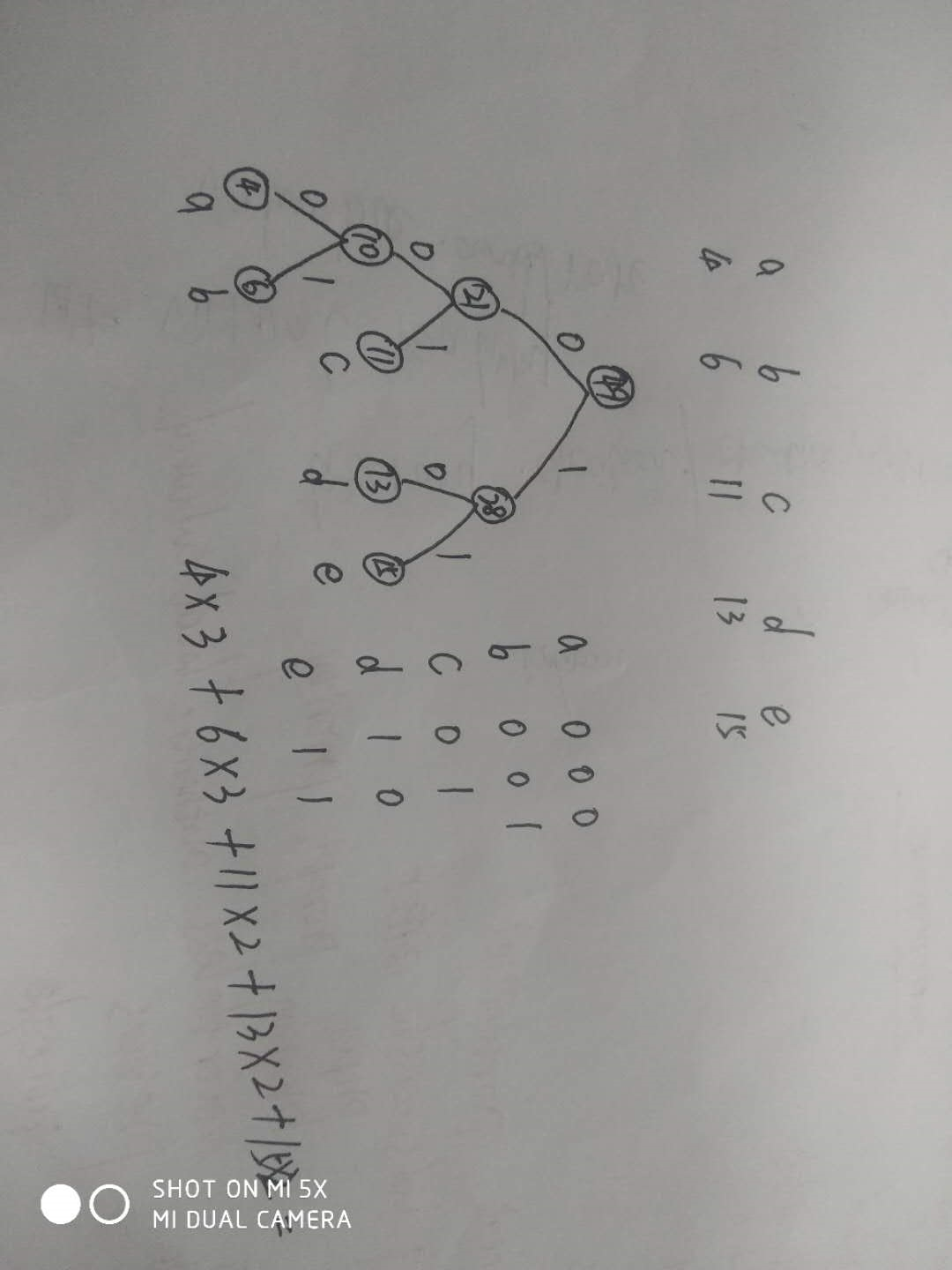用Python实现哈夫曼代码可参考：哈夫曼树的 Python 实现   或者：https://blog.csdn.net/qq_42098718/article/details/102809485
.选择排序:不稳定，时间复杂度 O(n^2)
插入排序:稳定，时间复杂度 O(n^2)
冒泡排序:稳定，时间复杂度 O(n^2)
堆排序:不稳定，时间复杂度 O(nlog n)
归并排序:稳定，时间复杂度 O(nlog n)
快速排序:不稳定，时间复杂度 最理想 O(nlogn) 最差时间O(n^2)
展开全文python
• 举例理解哈夫曼树，C语言实现哈夫曼

这篇文章存了一年了，因为最近需求颇多，在此分享出来。

本文举例帮助理解哈夫曼树的构造过程，并使用C语言实现了哈夫曼树的构造（代码可直接运行）。更多的基础知识不是本文的重点，请自行补充。

0.举例
要传输一则报文内容如下：
“AAAAAAAAAAAAAAABBBBBBBBBCCCCCCCCDDDDDDDDDDDDEEEEEEEEEEFFFFF”

请为这段报文设计哈夫曼编码。以下几点基本此实例进行，梳理了构造哈夫曼树的过程，C原因实现代码，算法时空效率分析。
1.构造哈夫曼树并得到哈夫曼编码的过程
第一步：计算各个字符的出现次数及频率
字符ABCDEF出现的次数159812105出现的频率0.2540.1530.1360.2030.1690.085
第二步：选定权值（出现次数及频率是等价的）
由于以频率作为权值和以出现的次数作为权值是等价的（频率同时扩大一定倍数即可得到出现的次数），故可以出现的次数作为哈夫曼树的权值。
第三步：开始构造哈夫曼树
整体构造哈夫曼树的思路如下：
从给定的n个权值中选出两个最小的权值，将这两个结点组成一棵新的二叉树，这棵二叉树的根结点的权值是这两个结点的权值之和。将上述最小的两个权值删去，将新的根节点的权值加入。重复1和2，直到所有的结点构成一棵二叉树（哈夫曼树）。
构造过程图：第四步：得到哈夫曼编码
按：左分支表示字符‘0’，右分支表示字符‘1’，即可从哈夫曼树中读取哈夫曼编码。 最终得到的哈夫曼编码如下：
原字符ABCDEF哈夫曼编码1011001100111010
2.代码实现上述哈夫曼编码
代码实现哈夫曼编码的总体思路是：
初始化输入的字符串，得到权值数组。将这个权值数组拿来构造哈夫曼树。从根节点开始读取哈夫曼编码。
其中，构造哈夫曼树的具体思路是：
先设计一个函数实现每次从权值数组中获取最小的两个权值。模拟上述构造哈夫曼树的过程进行构造。
具体实现代码（C语言）
#include<stdio.h>
#include<stdlib.h>
#include<string.h>

//哈夫曼树结点结构
typedef struct {
int weight;//权值
int parent;//父结点在数组中的位置下标
int left;//左孩子在数组中的位置下标
int right;//右孩子在数组中的位置下标
}HTNode, * HuffmanTree;

//存储哈夫曼编码
typedef char** HuffmanCode;

/*
函数作用说明：找到两个最小的权值并以w1，w2的形式传递
主要实现思路：先找到最先出现的两个未参与构建树的结点，再与后面所有未参与构建树的结点比较，更新minFirst，minSecond
该函数的时间复杂度：O(n) 只需遍历一次数组即可找到最小的两个权值
参数说明：
HT是哈夫曼树数组
n是HT有效的最后一个位置
w1和w2传递HT数组中权重值最小的两个结点在数组中的位置
*/
void GetTwoMin(HuffmanTree HT, int n, int* w1, int* w2){
int minFirst, minSecond;
//遍历
int index = 1;
//找到第一个未参与构建树的结点
while (HT[index].parent != 0 && index <= n) {
index++;
}
minFirst = HT[index].weight;
*w1 = index;
//找到第二个未参与构建树的结点
index++;
while (HT[index].parent != 0 && index <= n) {
index++;
}
//更新minFirst，minSecond
if (HT[index].weight < minFirst) {
minSecond = minFirst;
*w2 = *w1;
minFirst = HT[index].weight;
*w1 = index;
}else {
minSecond = HT[index].weight;
*w2 = index;
}
//一一比较
for (int i = index + 1; i <= n; i++){
//参与构建树，不考虑
if (HT[i].parent != 0) {
continue;
}
//更新minFirst，minSecond
if (HT[i].weight < minFirst) {
minSecond = minFirst;
minFirst = HT[i].weight;
*w2 = *w1;
*w1 = i;
}else if (HT[i].weight >= minFirst && HT[i].weight < minSecond) {
minSecond = HT[i].weight;
*w2 = i;
}
}
}

/*
函数作用说明：根据输入的权值数组构建哈夫曼树
主要实现思路：先分配空间并初始化，再依次选两个最小权值进行构建哈夫曼树
该函数的时间复杂度分析：嵌套主要发生在构建过程，设结点数为n，则时间复杂度为O(n*n)
参数说明：
HT是存储哈夫曼树的数组（传递的是地址）
w是存储结点权值的数组
n是结点个数
*/
void CreateHuffmanTree(HuffmanTree* HT, int* w, int n){
//一个字符，无需构建哈夫曼树
if (n <= 1) return;
//计算哈夫曼树总节点数（n只是叶子结点数）
int m = 2 * n - 1;
//分配HT的空间，下标从1开始
*HT = (HuffmanTree)malloc((m + 1) * sizeof(HTNode));
HuffmanTree ht = *HT;
// 初始化叶子结点
for (int i = 1; i <= n; i++){
(ht + i)->weight = *(w + i - 1);
(ht + i)->parent = 0;
(ht + i)->left = 0;
(ht + i)->right = 0;
}
//初始化其它结点
for (int i = n + 1; i <= m; i++){
(ht + i)->weight = 0;
(ht + i)->parent = 0;
(ht + i)->left = 0;
(ht + i)->right = 0;
}
//构建哈夫曼树的过程
for (int i = n + 1; i <= m; i++){
int w1, w2;
GetTwoMin(*HT, i - 1, &w1, &w2);
(*HT)[w1].parent = (*HT)[w2].parent = i;
(*HT)[i].left = w1;
(*HT)[i].right = w2;
(*HT)[i].weight = (*HT)[w1].weight + (*HT)[w2].weight;
}
}

/*
函数作用说明：根据哈夫曼树读取哈夫曼编码
主要实现思路：从叶子结点开始逆向的读取哈夫曼编码
该函数时间复杂度：O（n*log n）
参数说明：
HT是哈夫曼树
HC是存储结点哈夫曼编码的二维数组
n是哈夫曼树叶子结点的个数
*/
void HuffmanCoding(HuffmanTree HT, HuffmanCode* HC, int n) {
//为HC分配空间
*HC = (HuffmanCode)malloc((n + 1) * sizeof(char*));
//存放结点哈夫曼编码
char* codes = (char*)malloc(n * sizeof(char));
codes[n - 1] = '\0';
//逆序读取编码
for (int i = 1; i <= n; i++) {
//控制该结点哈夫曼编码的起始点
int begin = n - 1;
//当前结点在数组中的位置
int curr = i;
//当前结点的父结点在数组中的位置
int parent = HT[i].parent;
// 向根方向遍历，直到遍历到根
while (parent != 0) {
// 左孩子对应编码为0，右孩子编码为1
if (HT[parent].left == curr) {
codes[--begin] = '0';
}else {
codes[--begin] = '1';
}
//继续向根结点的方向遍历
curr = parent;
parent = HT[parent].parent;
}
//从begin开始，存放的是哈夫曼编码
(*HC)[i] = (char*)malloc((n - begin) * sizeof(char));
strcpy((*HC)[i], &codes[begin]);
}
free(codes);
}

//输出哈夫曼编码
void PrintHuffmanCode(HuffmanCode htable, char* zf, int n){
printf("该字符串中每个字符对应的哈夫曼编码如下 : \n");
for (int i = 1; i <= n; i++) {
printf("%c 的哈夫曼编码是 = %s\n", zf[i - 1], htable[i]);
}
}

int main(){
printf("请输入你需要编码的字符串(999字符以内)：\n");
char str;
gets_s(str);
int len = strlen(str);
//模拟ascii表
char asiTable;
int n = 0;
//初始化
for (int i = 1; i <= 128; i++) {
asiTable[i] = 0;
}
//将字符个数写入
for (int i = 0; i < len; i++) {
asiTable[str[i]]++;
}
//统计有效字符个数
for (int i = 1; i <= 128; i++) {
if (asiTable[i] != 0) {
n++;
}
}
//动态分配数组
int* w = (int*)malloc(n * sizeof(int));
char* f = (char*)malloc((n + 1) * sizeof(char));
int index = 0;
//得到权值数组和对应的字符
for (int i = 1; i <= 128; i++) {
if (asiTable[i] != 0) {
w[index] = asiTable[i];
f[index] = (char)i;
index++;
}
}
HuffmanTree huffmanTree;
HuffmanCode huffmanCodes;
CreateHuffmanTree(&huffmanTree, w, n);
HuffmanCoding(huffmanTree, &huffmanCodes, n);
PrintHuffmanCode(huffmanCodes, f, n);
return 0;
}

运行结果图：3.算法效率分析
时间复杂度：
假设输入的字符串长度为k，不同字符数为n，时间复杂度的分析如下：
整体分析： 整个程序主要消耗的时间在于构造哈夫曼树和得到哈夫曼编码，其中构造哈夫曼树所消耗的时间最多，故总的时间复杂度为O(n^2)对于处理输入字符串的数据部分：主要消耗的时间是统计不同字符出现的次数，此处的时间复杂度为：O(k)对于构造哈夫曼树的部分：主要消耗的时间是循环构造树，其中每次循环构造树时，需要先选取两个最小权值，这个选取是线性的，时间复杂度为O(n)，因此总的时间复杂度为O(n^2)对于得到哈夫曼编码的部分：主要消耗的时间是循环获取编码，其中每次循环获取编码时，需要向上遍历到根节点，时间复杂度为O(log n)，因此总的时间复杂度为O(n*log n)对于输出哈夫曼编码的部分：线性的输出，时间复杂度为O(n)
空间复杂度：
假设输入的字符串长度为k，不同字符数为n，空间复杂度的分析如下：
整体分析： 整个程序中所用到的空间有：ascii表占128，w数组占n，f数组占n+1，HT数组占2n，HC数组占nlogn。其中HC数组所消耗的空间最多，因此，总的空间复杂度为**O(nlog n)**对于处理数据部分： 主要为n数组和f数组所消耗的空间，为O(n)对于构造哈夫曼树部分： 主要为哈夫曼树所消耗的空间，为O(n)对于得到哈夫曼编码部分： 主要为存储哈夫曼编码的HC数组所消耗，为O(n*log n)

ATFWUS POST：2021-06-20 WRITE：2020-07-01


展开全文哈夫曼树 C语言 二叉树
• 文章目录哈夫曼树和哈夫曼编码关于编码哈夫曼树哈夫曼编码构造哈夫曼树 哈夫曼树和哈夫曼编码 关于编码 常用编码方式 等长码：每个字符对应码字的码长都一样，例如ASCII码表中的128个字符可以用7位码长的01位串...


文章目录
哈夫曼树和哈夫曼编码关于编码哈夫曼树哈夫曼编码构造哈夫曼树

哈夫曼树和哈夫曼编码
关于编码
常用编码方式 等长码：每个字符对应码字的码长都一样，例如ASCII码表中的128个字符可以用7位码长的01位串表示(

2

7

=

128

2^7=128

）。思考 能不能用不等长的编码方式来编码字符，出现次数较多的字符用较短的码长的码字来存储，出现次数较少的字符用较长的码长的码字来存储，使得存储所有字符用更少的空间？问题
如何进行不等长编码？ 想要采用不等长编码的方式，那么应该怎么构造一个字符与不同码长的码字之间的一一对应的关系？最长的码字的码长应该是多少？最短的码字的码长有应该是多少？  如何避免不等长编码方式的二义性？ 例：假定以下字符采用下面的不等长编码方式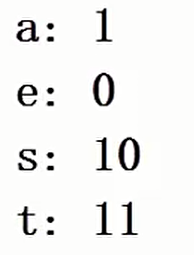那么1011表示的是哪个字符串的编码？ 可以看出，1011可以表示aeaa、aet和st。这就产生了编码的二义性。所以编码时一定要是前缀码，这样才能避免编码的二义性。
前缀码(Prefix Code): 任何字符的编码都不是另一个字符的前缀。  解决 利用哈夫曼树来构造前缀码。
哈夫曼树
带权路径长度(WPL) 设二叉树有n个叶子节点，每个叶子节点带有权值

W

k

W_k

，从根节点到每个叶子节点的长度为

L

k

L_k

，则每个叶子节点的带权路径长度之和就是

W

P

L

=

∑

k

=

1

n

W

k

L

k

WPL=\sum_{k=1}^{n}{{W_k}{L_k}}

哈夫曼树（最优二叉树） WPL最小的二叉树。 例：写一个程序将百分制的考试成绩转换成五分制的成绩。60分以下是等级1，60到70分是等级2，70到80分是等级3，80到90分是等级4，90到100是等级5。 学生成绩分布概率如下：
分数段0-5960-6970-7980-8990-100比例0.050.150.400.300.10对比以下两种程序结构： 结构1：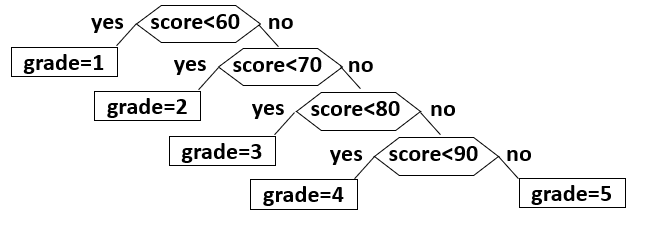结构二：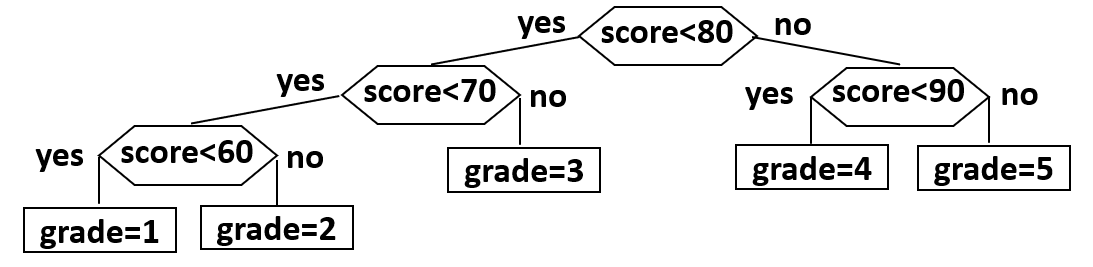结构1的平均查找速率（这棵判定树的WPL）：

0.05

×

1

+

0.15

×

2

+

0.4

×

3

+

0.3

×

4

+

0.1

×

4

=

3.15

0.05 \times 1+0.15 \times 2+0.4 \times 3+0.3 \times 4+0.1 \times 4=3.15

结构2的平均查找速率（这棵判定树的WPL）：

0.05

×

3

+

0.15

×

3

+

0.4

×

2

+

0.3

×

2

+

0.1

×

2

=

2.2

0.05 \times 3+0.15 \times 3+0.4 \times 2+0.3 \times 2+0.1 \times 2=2.2

可以看出，将结构1、2看成一棵判定树，每个等级就成了树的叶子节点，叶子节点的权值就是其成绩分布概率，而需要判断的个数就是该节点到树根的距离。这样，平均查找速率就是这棵树的WPL。不同的结构，树的WPL不同，而WPL最小的那棵树就是哈夫曼树。
哈夫曼编码
利用二叉树构造前缀码
左右分支分别代表0和1；字符只在叶节点上。 例：对字符a、u、x和z四个字符进行编码。
等长码：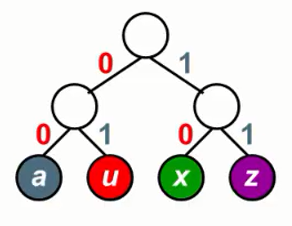a：00 u：01 x：10 z：11不等长码：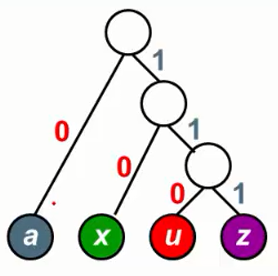a：0 u：110 x：10 z：111 可以看到利用二叉树可以构造出前缀码，使得编码没有二义性。  利用哈夫曼树编码使得存储空间最小 例：一段字符由a、u、x和z组成，其中a出现4次，u出现1次，x出现2次，z出现一次。怎样的编码方式才能使这段字符存储空间最小？ 首先，我们可以计算利用等长码编码所用到的存储空间：

C

o

s

t

=

2

×

4

+

2

×

1

+

2

×

2

+

2

×

1

=

16

Cost=2 \times 4+2 \times 1+2 \times 2+2 \times 1=16

我们可以看到，存储空间的计算就相当于将字符出现的频率当作叶子结点的权值，每个字符码字的码长当作根节点到叶子节点的长度，所用存储空间就是这棵树的WPL。所以构造一棵哈夫曼树用来编码，所用的存储空间最小，如上不等长编码就是一棵哈夫曼树，其所用到的存储空间为：

C

o

s

t

=

1

×

4

+

3

×

1

+

2

×

2

+

3

×

1

=

14

Cost=1 \times 4+3 \times 1+2 \times 2+3 \times 1=14

构造哈夫曼树
步骤：
将所有叶子节点的权值从小到大排列；取出权值最小的两个节点，构成一棵二叉树，他们是两个子节点，他们的父节点的权值等于他们权值的和。在将他们的父节点权值与剩下的叶子节点的权值排序；重复这个过程，直到序列中只有一个节点的权值  过程示例：
将所有叶子节点的权值从小到大排列：取出最小两个节点构成一棵二叉树，他们的权值和加入序列并重新排序；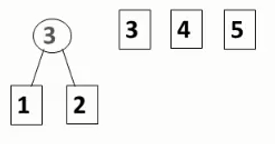重复第二步：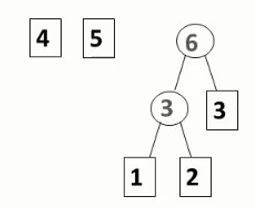重复第二步：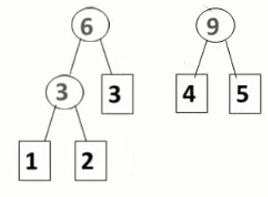直到序列中只有一个权值：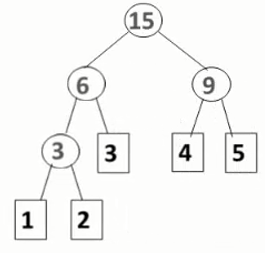依照这个步骤，一棵哈夫曼树就构造好了  代码分析
哈夫曼树节点表示 typedef struct TreeNode{
int Weight;//节点的权值
struct TreeNode *Left;//指向左子树的指针
struct TreeNode *Right;//指向右子树的指针
}HuffmanTreeNode,*HuffmanTree;
  代码执行过程中，每次都要取权值序列中最小的两个节点出来，所以每次都要进行一次排序；不过还有一种方法就是利用最小堆的特性，进行两次取出操作，取出最小值，然后插入新的权值。相较于前者，后者的时间复杂度比较低。（堆的一些方法与实现）  具体代码（C语言） //构造哈夫曼树
//所有权值都存在HuffmanTreeNode类型的数组中，HT指向该数组，数组元素个数为Size
HuffmanTree BuildHuffmanTree(HuffmanTree HT, int Size)
{
MinHeap H = NULL;//构建最小堆
HuffmanTree HTRoot = NULL;
int i = 0,size;

//用数组构建一个最小堆
H = BuildHeap(MaxSize, HT, Size);

size = H->Size;//用size暂存堆中元素个数，否者在插入和删除过程中，H->Size会改变
for (i = 0; i <size-1; i++)
{
HTRoot = (HuffmanTree)malloc(sizeof(HuffmanTreeNode));//构建一个新的节点

HTRoot->Left = DeleteHeap(H);
HTRoot->Right = DeleteHeap(H);//新节点左右子树指针分别指向权值最小的两个节点
HTRoot->weight = (HTRoot->Left->weight) + (HTRoot->Right->weight);//新节点权值为两个权值最小节点的权值的和

InsertHeap(H, HTRoot);//将新节点插入堆中
}

HTRoot = DeleteHeap(H);//将HTRoot指向堆中最后的一个节点，作为哈夫曼树的根

//释放堆的空间
DestroyHeap(H);

return HTRoot;//返回哈夫曼树的根节点
}
  
展开全文数据结构
• 最近多媒体布置了一个哈夫曼树的压缩算反的题目。然后我觉得我里面用了不少...然后我用哈夫曼树去进行编码复杂度我也把它降成了O(n)这两部都是线性就能解决问题。 最后一个解码。没办法只能变成O(KN),K是哈夫曼java 多媒体 算法
• 哈夫曼编码的简单实例，对a-e 5个字母进行编码，随机生成0-30个 a-e 的字母序列，对其进行编码和解码。带可视化界面。
• ## 哈夫曼树与哈夫曼编码及代码实现

千次阅读 多人点赞 2020-03-18 12:43:30
哈夫曼树与哈夫曼编码的理解 数据压缩 含义 通过对数据重新的编码，减少数据占用的空间存储；使用的时候再进行解压缩，恢复数据的原有特性。 类别 无损压缩——压缩过程没有数据丢失，解压得到原有数据特性。 有损...二叉树 数据结构 算法 链表
• 问题描述：利用哈夫曼编码进行信息通讯可以大大提高信道的利用率，缩短信息传输时间，降低传输成本。但是，这要求在发送端通过一个编码系统对待传输数据预先编码；在接受端将传来的数据进行译码。对于双工信道（即...
• 06哈夫曼编码 复杂度： O(nlogn) 算法： #coding=utf-8 """ 算法：哈夫曼编码 作者：lph-China 时间：2019/7/15 """ def huffman(freq): h = [] for a in freq: heappush(h, (freq[a], a)) while ...高效算法 python
• 哈夫曼编码属于最优编码，可以构造编码长度最短的编码方案。哈夫曼编码和各个字符出现的概率有关。假设要传送一组字符串为ABACCDA，其中A出现3次，B出现1次，C出现2次，D出现1次，根据哈夫曼编码，编码结果为A：0，B...java
•   这篇博客主要讲解如何用java实现哈夫曼编码（Huffman）。 概念   首先，我来简单说一下哈夫曼编码（Huffman），它主要是数据编码的一种方式，也是数据压缩的一种方法，将某些特定的字符转化为二进制字符，并...huffman java
•数据结构 算法 二叉树
• @[TOC] C语言哈夫曼编码 哈夫曼编码 C 实现 思考 之前做过一个编码，解码的，都是先有一个密码本。 编码：输入明文的字符串根据码本编码， 解码：输入密文的密码，跟局码本转换为明文的字符串。 所以第一件事应该...c语言
• ## 哈夫曼编码详解(C语言实现)

万次阅读 多人点赞 2018-12-21 21:28:43
关于算法我们可以从如下几部分分析：算法的逻辑结构、算法的存储结构、以及算法本身考虑，同时也需要考虑时间和空间的复杂度，关于哈夫曼编码，我们尝试着这几方面分析这个问题 逻辑结构 我们采用的是树这种的逻...算法 自我总结
• 哈夫曼树和哈夫曼编码 二叉树及二叉树的遍历 二叉树，从名字上就能理解，两个叉的树，每个父节点只有左子节点和右子节点。 ![这是一棵二叉树](/home/pidan/图片/2018-12-06 15-29-08 的屏幕截图.png) 关于...
• 哈夫曼树与哈夫曼编码 什么是哈夫曼树 判定树 考虑学生成绩的分布的概率 查找效率 修改判定树 根据结点不同的查找概率构造更有效的搜索树 哈夫曼树的定义 带权路径长度（WPL）：设二叉树有n个叶子节点，每个...二叉树 数据结构 算法
• 本程序是VS2010下的源程序，可直接运行。...本程序实现了通过读取文件中关于字符的相关说明数据来初始化相关变量，最后采用贪心算法的思想编程实现哈夫曼编码的求解。最终输出各个字符的哈弗曼编码值。
• ## 哈夫曼编码的实验报告

千次阅读 多人点赞 2020-11-28 19:49:24
哈夫曼编码实验报告 一、实验目的         通过哈夫曼编、译码算法的实现，巩固二叉树及哈夫曼树相关知识的理解掌握，训练学生运用所学知识，解决实际问题的能力。 二.实验...
• 9. 哈夫曼树和哈夫曼编码9.1 什么是哈夫曼树9.2 哈夫曼树的构造9.3 哈夫曼编码9.4 最小堆实现哈夫曼树 9. 哈夫曼树和哈夫曼编码 9.1 什么是哈夫曼树   给定N个权值作为N个叶子结点，构造一棵二叉树，若该树的带权...数据结构
• 目录 一、什么是哈夫曼树（Huffman Tree） 1.1 哈夫曼树的定义 二、哈夫曼树的构造 2.1 哈夫曼树的特点 三、哈夫曼编码 3.1 使用二叉树编码 3.2 使用哈夫曼树编码 ...
• 实现Huffman编码是用贪心算法来实现的，。实现Huffman最好的数据结构时优先级队列...但是这里的时间复杂度却提高了，因为操作set的选择时，时间复杂度时lgn,但是随着选择的，选择“未被访问的最高优先级的两个元素（flC 算法
• 自适应哈夫曼编码由于其良好的实时性,特别适合于通信系统等对速度要求高的场合 。为此提出一种新的自适应哈夫曼编码算法,它利用符号到达...与原有的 V算法相比,有效降低了编码复杂度,占用存储资源较少,易于硬件实现 。
• 哈夫曼树的本质就是在k进制下找非前缀总长最小编码，非前缀使得解码唯一，总长最小使储存和传输都更优。 对每个信息节点都有一个出现次数作为权值，权值乘上长度就是它对编码总长的贡献，问题就是要使得所有权值乘...
• 哈哈夫曼树一、初始化函数init()二、合并操作unite()三、查询树根函数find()四、改进后的合并操作unite()五、改进后的find()六、判断是否属于同一集合same()并查集的复杂度并查集的实现代码 哈夫曼树 并查集是一种...数据结构 二叉树 霍夫曼树 算法 c++...